Courses

# Single Stage Amplifiers Electrical Engineering (EE) Notes | EduRev

## Electronic Devices

Created by: Cstoppers Instructors

## Electrical Engineering (EE) : Single Stage Amplifiers Electrical Engineering (EE) Notes | EduRev

The document Single Stage Amplifiers Electrical Engineering (EE) Notes | EduRev is a part of the Electrical Engineering (EE) Course Electronic Devices.
All you need of Electrical Engineering (EE) at this link: Electrical Engineering (EE)

1 Introduction

V-I characteristics of an active device such as BJT are non-linear. The analysis of a non- linear device is complex. Thus to simplify the analysis of the BJT, its operation is restricted to the linear V-I characteristics around the Q-point i.e. in the active region. This approximation is possible only with small input signals. With small input signals transistor can be replaced with small signal linear model. This model is also called small signal equivalent circuit.

1.2 Two –Port  Devices and Network Parameters

Small signal low frequency transistor Models:

All the transistor amplifiers are two port networks having two voltages and two currents. The positive directions of voltages and currents are shown in fig. 1.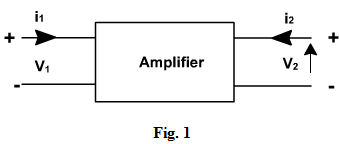A two-port network is represented by four external variables: voltage Vand current  Iat the input port, and voltage Vand current  I2 at the output port, so that the two-port network can be treated as a black box modeled by the relationships between the four variables,V1,V2, I1,I2 . Out of four variables, two can be selected as independent variables and the other two as dependent variables. The dependent variables can be expressed in terms of independent variables. This leads to various two port parameters out of which the following three are important:

1.      Impedance parameters (z-parameters)

3.      Hybrid parameters (h-parameters)

1 z-parameters

A two-port network can be described by z-parameters as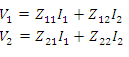In matrix form, the above equation can be rewritten as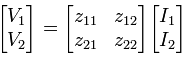Where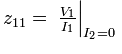Input impedance with output port open circuited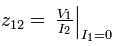Reverse transfer impedance with input port open circuited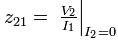Forward transfer impedance with output port open circuited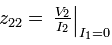Output impedance with input port open circuited

Y-parameters

A two-port network can be described by Y-parameters as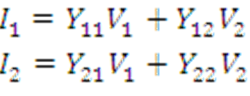In matrix form, the above equation can be rewritten as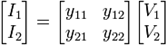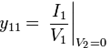Input admittance with output port short circuited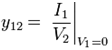Reverse transfer admittance with input port short circuited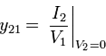Forward transfer admittance with output port short circuited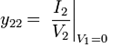Output admittance with input port short circuited

Hybrid parameters (h-parameters)

If the input current I1 and output voltage V2 are taken as independent variables, the dependent variables V1 and I2 can be written as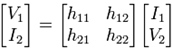Where h11, h12, h21, h22 are called as hybrid parameters.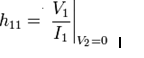Input impedance with o/p port short circuited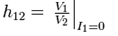Reverse voltage transfer ratio with i/p port open circuited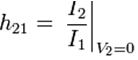Forward voltage transfer ratio with o/p port short circuited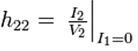output impedance with i/p port open circuited

Offer running on EduRev: Apply code STAYHOME200 to get INR 200 off on our premium plan EduRev Infinity!

,

,

,

,

,

,

,

,

,

,

,

,

,

,

,

,

,

,

,

,

,

;